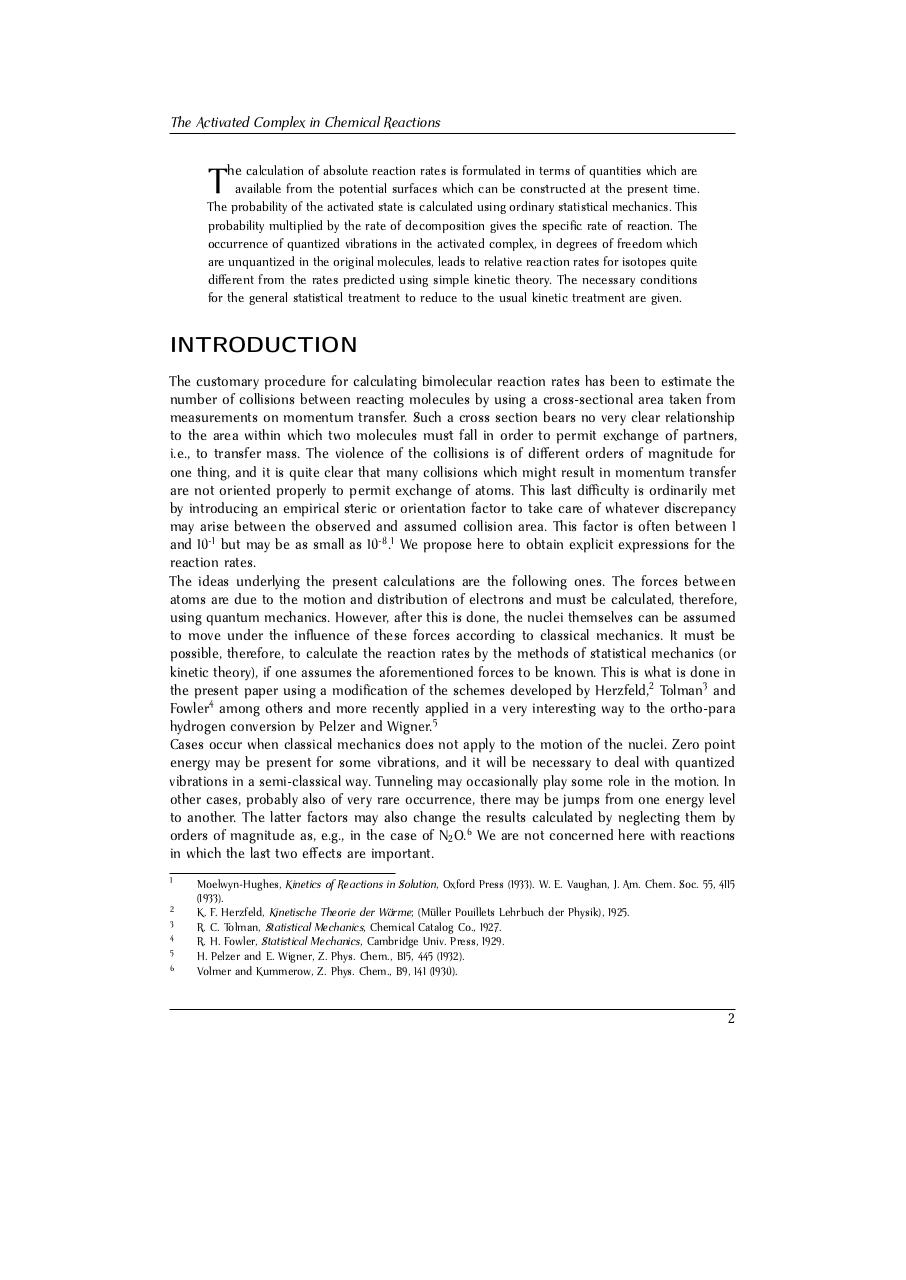# Henry Eyring. The Activated Complex in Chemi.pdfPage 1 2 3 4 5 6 7 8 9 10 11 12 13

#### Text preview

The Activated Complex in Chemical Reactions
he calculation of absolute reaction rates is formulated in terms of quantities which are

T

available from the potential surfaces which can be constructed at the present time.
The probability of the activated state is calculated using ordinary statistical mechanics. This
probability multiplied by the rate of decomposition gives the specific rate of reaction. The
occurrence of quantized vibrations in the activated complex, in degrees of freedom which
are unquantized in the original molecules, leads to relative reaction rates for isotopes quite
different from the rates predicted using simple kinetic theory. The necessary conditions
for the general statistical treatment to reduce to the usual kinetic treatment are given.

INTRODUCTION
The customary procedure for calculating bimolecular reaction rates has been to estimate the
number of collisions between reacting molecules by using a cross-sectional area taken from
measurements on momentum transfer. Such a cross section bears no very clear relationship
to the area within which two molecules must fall in order to permit exchange of partners,
i.e., to transfer mass. The violence of the collisions is of different orders of magnitude for
one thing, and it is quite clear that many collisions which might result in momentum transfer
are not oriented properly to permit exchange of atoms. This last difficulty is ordinarily met
by introducing an empirical steric or orientation factor to take care of whatever discrepancy
may arise between the observed and assumed collision area. This factor is often between 1
and 10-1 but may be as small as 10-8 .1 We propose here to obtain explicit expressions for the
reaction rates.
The ideas underlying the present calculations are the following ones. The forces between
atoms are due to the motion and distribution of electrons and must be calculated, therefore,
using quantum mechanics. However, after this is done, the nuclei themselves can be assumed
to move under the influence of these forces according to classical mechanics. It must be
possible, therefore, to calculate the reaction rates by the methods of statistical mechanics (or
kinetic theory), if one assumes the aforementioned forces to be known. This is what is done in
the present paper using a modification of the schemes developed by Herzfeld,2 Tolman3 and
Fowler4 among others and more recently applied in a very interesting way to the ortho-para
hydrogen conversion by Pelzer and Wigner.5
Cases occur when classical mechanics does not apply to the motion of the nuclei. Zero point
energy may be present for some vibrations, and it will be necessary to deal with quantized
vibrations in a semi-classical way. Tunneling may occasionally play some role in the motion. In
other cases, probably also of very rare occurrence, there may be jumps from one energy level
to another. The latter factors may also change the results calculated by neglecting them by
orders of magnitude as, e.g., in the case of N2 O.6 We are not concerned here with reactions
in which the last two effects are important.
1
2
3
4
5
6

Moelwyn-Hughes, Kinetics of Reactions in Solution, Oxford Press (1933). W. E. Vaughan, J. Am. Chem. Soc. 55, 4115
(1933).
K. F. Herzfeld, Kinetische Theorie der Wärme; (Müller Pouillets Lehrbuch der Physik), 1925.
R. C. Tolman, Statistical Mechanics, Chemical Catalog Co., 1927.
R. H. Fowler, Statistical Mechanics, Cambridge Univ. Press, 1929.
H. Pelzer and E. Wigner, Z. Phys. Chem., B15, 445 (1932).
Volmer and Kummerow, Z. Phys. Chem., B9, 141 (1930).

2